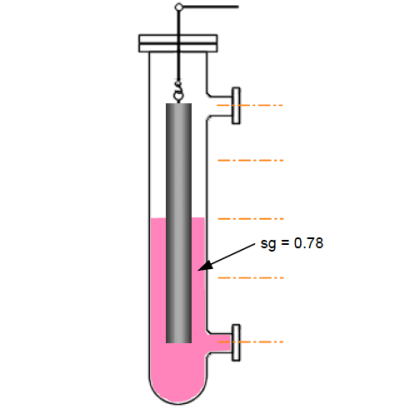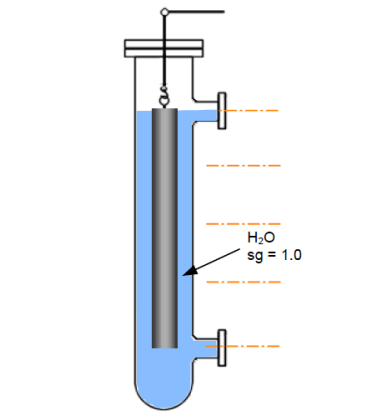Home » Displacer Level Measurement Calculations

Displacer Level Measurement Calculations

Displacer Level Measurement Calculations

Problem 1 :-

Calculate the apparent weight of the displacer.true weight of displacer = 0.25 × 490 = 122.5 lbs

volume of oil displaced = 0.25 × 0.5 = 0.125 cubic feet

weight of oil displaced = (0.125 × 0.78 × 63) lbs = 6.143 lbs

∴ Apparent weight of displacer = true weight − displaced weight

Apparent weight of displacer = 122.5 − 6.143 lbs = 116.36 lbs

Problem 2 :-

Calculate the following;1:- the maximum and minimum weights of the displacer.2:- the maximum range of level able to be sensed, if the diameter of the displ. is 2 inch.true weight of displacer = 0.1 × 490 lbs = 49 lbs

volume of water displaced = 0.1 cubic feet

weight of water displaced = 0.1 × 63 lbs = 6.3 lbs

∴ min. weight of the displacer = 49 − 6.3 lbs = 42.7 lbs

∴ max. weight of the displacer = 49 lbs

volume of the sensor = πr2L

Note : π = 3.142, 1 in = 1/12 ft = 0.0833ft

volume of the sensor ⇒ 0.1 ft3 = 3.142 × (0.0833)2 × L

0.1 ft3 = 0.0218 × L

hence, the length of displacer, L = 0.1 / 0.0218 = 4.587 ft , or 55 inches

Problem 3 :-

1:- the diameter of the displacer. 2:- the displacer weight in air, oil & water. 3:- the “0%” and “100%” weights, if the 50% interface level is set at the displacer’s mid-point, and the span is 0.5 metres.

The displacer is assumed to be totally covered with oil or water.volume of the sensor = πr2L

1000 c.c.s = 3.142 × r2 × 100 cm

thus, we now have, r2 =  1000 / (3.142 x 100 ) = 3.183 cm2

∴ the radius of displacer, r = Sqrt (3.183) cm = 1.78 cm

hence, the diameter of displacer = 1.78 × 2 cm = 3.56 cm

displacer’s weight in air = density × volume

Note that volume unit has to be inconsistent with density unit 1 ccs = 10−6 m3

= 8000 kgm−3 × (1×103/106) m3 = 8.0 kg

displacer’s weight in oil = (weight in air) − (weight loss in oil)

= 8.0 – (0.5 × 1 × 0.78) kg = 7.61 kg

displacer’s weight in water = (weight in air) − (weight loss in water)

= 8.0 – (0.5 × 1 × 1) kg = 7.50 kg

at 0% interface level, the displacer’s length will be covered 25% with water and 75% with oil. Hence, the displacer’s total weight will be made up of 75% of its weight in oil and 25% weight in water;

∴ displacer weight at 0% = (0.75 × 7.61) + (0.25 × 7.5) = 7.582 kg

at 50%, the displacer’s total weight will be made up of 50% weight in water, and 50% weight in oil;

∴ displacer weight at 50% = (0.5 × 7.61) + (0.5 × 7.5) = 7.555 kg

at 100%, the displacer’s total weight will be made up of 75% weight in water, and 25% weight in oil;

∴ displacer weight at 50% = (0.25 × 7.61) + (0.75 × 7.5) = 7.527 kg

Article Source : N. Asyiddin

Articles You May Like :

Torque Tube Level Measurement

Conductive Level Switch Principle

Ultrasonic Level Switch Principle

Level Measurement with wet leg

DP Level Transmitter Calibration